# Types

Programs manipulate values according to their type. There is more to the study of types than you might think.

## Why Types?

Our very intuition makes the concept of types inescapable.

We have an intuition that integers are different from strings which are different from people which are different from books which are different from planets which are, you get the idea. These differences arise from behavior: You can open a socket, but not an integer; you can request the parents of a person, but not a dictionary; you can push an object onto a stack, but not onto character. Therefore:

A value’s type constrains the way it may be used in a program.

More philosophically:

Types impose constraints on what we can and cannot say.

More formally:

A type consists of a set of values and a set of allowable operations.

## Examples

What are some types you know about from previous programming practice? Here are some you might have seen, Keep in mind, as you go through this list, that types are characterized by their operations (more so than their “values”):

• Empty Types, which are types that cannot have any values. An empty type is also called a bottom type. In some languages, the bottom type is called void; in others it is called never; and still other names may be used.
• boolean, the type containing the values true and false, with operations such as and, or, xor, and not.
• atom: The type of named symbols. Atoms just have name, and that’s it. You don’t really operate on them (other than testing them for equality perhaps); you just pass them around. They just exist. Some languages call the type of atoms symbol.
• Numeric types, with operations such as plus, minus, multiply, divide, remainder, modulo, abs, sign, sin, cos, tan, and on and on and on. Numeric types can be integral, fixed-point, floating point, or complex; bounded or unbounded; signed or unsigned. Examples include: number, int8, int16, int32, int64, int128, int, uint8, uint16, uint32, uint64, uint128, uint, bigint, fixed, float32, float64, float128, complex64, complex128, ratio, decimal, bigdecimal.
• char: The type of “characters”, i.e., units of textual information. Exactly what a character is might differ from language to language.
• Collection Types, with operations such as size, is_empty, contains, add, remove, retain, clear.
• Set Types, which are unordered collections of unique elements.
• Sequence Types. So many of these, including arrays, lists, stacks, queues, dequeues, priority queues, blocking queues. Even the famous string is a kind of sequence, but there’s not a lot of agreement about what exactly strings are sequences of. Are they sequences of bytes? character codes? code points? unicode scalars? graphemes? String types are generally broken. You don't need them.
• Mapping Types, types that maps keys to values. If the keys are unique we call them maps or dictionaries; if keys do not have to be unique we call them multimaps. Normally the keys are unordered, but ordered maps or those that are automatically iterated by in key-order (assuming keys are comparable).
• Singleton types, which are types that only have one value in them. The operations on that individual value vary, of course. Sometimes these are called unit types or literal types (in the case where the type name and the value name are the same, as in TypeScript).
• Sum Types. The type $T_1 + T_2$ consists of all the values of $T_1$ plus all the values of $T_2$. Sums are often called unions. If the alternatives are labeled (named), we get tagged unions.
• Product Types, The type $T_1 \times T_2$ consists of all pairs $(x, y)$ where $x$ has type $T_1$ and $y$ has type $T_2$. In general you can have products of any size: $T_1 \times T_2 \times \ldots \times T_n$. If $n=0$ you have what’s sometimes called the unit type: the type of only one value. In general, product types are called tuple types. If the components are labeled (named), we get records.
• Routines, which can be subroutines or coroutines. Operations on routines include invoke and invokeLater. Other terms for invoke include call and apply (from the idea of function “application” — “applying” a function to its argument(s)).
• Function types, computational mappings from arguments to results. We can classify functions in many ways: there are predicates, which return booleans, consumers, that accept arguments but do not return results, suppliers, which take no arguments but return results. In some languages, the number, order, names, and types of parameters are part of the function type, as is the function’s return type.
• Processes, Threads, and Tasks, which are things that run asynchronously. Generally these things send messages to each other, but shared memory is sometimes used. They are generally created with a spawn operation. Internally they use send and receive to communicate.
• The type type, whose members are...types!
• Top types, that contain every single possible value. In TypeScript, the top type is unknown. Other languages call it any (though “any” generally has a completely different meaning in gradually-typed languages, so be careful!)
Exercise: Spend some time thinking about the behaviors of each of the types above.

## Classifying Types

It is tempting to try to put types into a hierarchy, or at least a directed acyclic graph: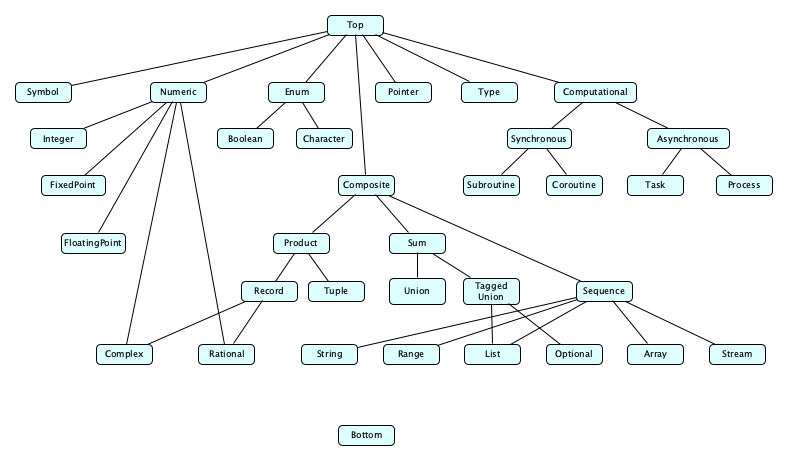But it’s actually difficult to this because there are so many other ways to classify type that defy hierarchy. In fact, there’s this whole idea of classifying types into something called typeclasses, which are things like this:

A type is equatable, or is an eqtype, if values of the type can be tested for equality (==).

• In some languages, all types are equatable because most of the types have reference semantics. In Haskell, however, functions, for example, are not equatable.

A type is comparable (a.k.a. ordered) if values of the type can be compared with < (and often also with <=, >, and >=, and often <=>).

• Generally (at least in the vast majority of languages), if you make your own class, it won’t be comparable be default.

A type is bounded if there is a minimum value of the type and a maximum value of the type.

• Fixed-size integers and simple enumerations are bounded.

A type is showable, or is a showtype if values of the type can be converted to strings.

• Functions and binary streams are generally not showable.

A type is callable if values of its type can be called on, or applied to, other values.

• Functions are callable. In Python, so are types!

A type is enumerable if it has successor and predecessor operations on its values.

• Integers are enumerable, as are characters (enumerated via their code points). Note this definition does not apply to languages with very complex enum things, like Swift. Those concepts are completely different.

A type is monoidal, or is a monoid, if it has an associative composition operator, and an identity operator for the composition.

• Concrete example: Functions! They have function composition and the identity x => x (JS notation).
• Abstractly: $\exists e, \bullet:\; \forall a, b, c: a \bullet (b \bullet c) = (a \bullet b) \bullet c \wedge a \bullet e = e \bullet a$

A type is monadic, or is a monad, if values of the type “wrap” underlying values in some way (e.g. optional, list), and there is an operator to wrap a value, and an associative composition operator on functions that unwrap a value and do something to it to produce a new wrapped value, such that the wrapper is an identity for the composition.

Typeclasses in Practice

Languages vary greatly in their treatment of typeclasses. Haskell has a distinguished typeclass concept (called a class. Swift implements them via protocols, and other languages just use their own interface or mixin construct.
Exercise: Find the built-in Swift protocols for equatables and comparables. How do they work? Craft an example that uses them.

## A Type Algebra

We can arrange types by building them up algebraically, similar to how sets are built up, for example:

Kind of TypeNotationNotes
Empty$\perp$Type with no members at all. A bottom type (meaning it is a subtype of all types). Sometimes called Void.
Singleton$x$Type with only a single member. Examples:
 null type containing only the value null true type containing only the value true "hello" ttype containing only the value "hello" 5 type containing only the value 5 blue type containing only the value blue
Sum$T_1\;|\;T_2\;|\,...|\;T_n$
or
$x_1: T_1\;|\,...|\;x_n: T_n$
Also written $T_1 \cup T_2$ or $T_1 + T_2$. If unlabeled, generally called a Union. If labeled, generally called a Tagged Union. Examples:
 true | false red | green | blue string | null int | bool | float 1 | 2 | 3 | 4 | 5 | 6 circle: float | rectangle: float × float enum { circle: Float; rectangle: (Float, Float) }
Product$(T_1, T_2, ... T_n)$
or
$(x_1: T_1,..., x_n: T_n)$
Also written $T_1 \times T_2$. If unlabled, generally called a Tuple. If labeled, generally called a Record or Struct. Examples:
 bool × int (Bool, Int) () x: int × y: int struct { x: int; y: int; }
Sequence$T*$a.k.a. Array or List. Examples:
 [Int] Swift []float64 Go bool list SML List Java
Function$T_1 \rightarrow T_2$A function from $T_1$ to $T_2$. Use $\perp$ or a product type for $T_1$ to simulate zero or multiple arguments, and $\perp$ or a product type for $T_2$ to simulate zero or multiple return values. Examples:
 Int -> Int Bool* -> Int × String Int × Int × String -> Int Float → Void
Top$\top$All values are a member of this type. The “type of everything.” A top type (meaning all types are a subtype of it). Examples:
 unknown TypeScript interface{} Go BasicObject Ruby object Python

This arrangement actually works pretty well, as it covers most everything we need:

• The boolean type is really just true | false for singleton types true and false.
• A string type is just a sequence of some underlying elements, maybe bytes, maybe character codes, maybe graphemes.
• An optional type is just T | null for any type T and singleton type null. This is often abbreviated T? or Optional<T>.
• Are sequence types really primitive? Seems like we can just write $T* = T \cup (T \times T) \cup (T \times T \times T) \cup \ldots$, but the abbreviation is simpler, of course. Be pragmatic.
• Many statically typed languages have a generic Either type, a tagged union with labels such as left and right, or a similarly structured tagged union called Result with labels success and failure. The latter is commonly used for returning, rather than throwing errors.
• Bottom types such as Never can be used for the return type of a function that does not return, either because it enters an infinite loop or throws an error.
Exercise: In your own words, describe the difference between a sum type and a product type.

In case you haven’t seen singleton or union types before, you might not have seen TypeScript.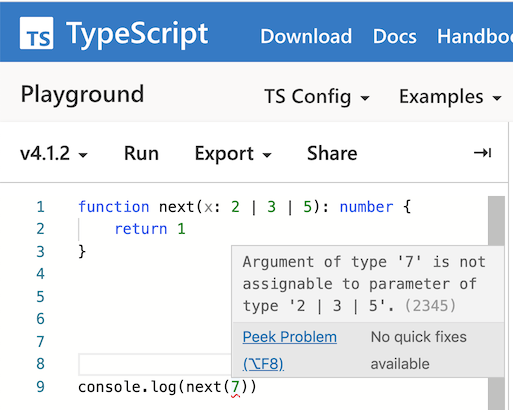## Type Systems

In programming language theory, a language’s type system answers questions such as:

1. Is the set of types fixed, or can you create new ones?
2. Are types extra-lingual, or are they themselves values? If values, are they first-class? Also if values, do they themselves belong to one of possibly many types or is there just one type? If not, can they be categorized into different, um, say, typeclasses?
3. What exactly are the types of the language, and how are new types built?
4. How do we infer the type of an expression (gets tricky with variables)
5. How do we know when two types are exactly the same?
6. How do we know when two types are compatible?
7. What do we do when expressions are used in a way inconsistent with their type?

## Fixed Type Systems

Some languages predefine the set of types and do not let you define new ones. These include:

• JavaScript: The only types are these eight: Undefined, Null, Boolean, String, Number, BigInt, Symbol, Object
• Lua: The only types are these eight: nil, boolean, number, string, function, thread, userdata, table.
• Erlang: The only types are: atom, integer, float, binary, ref, pid, port, function, list, tuple, map.

JavaScript really does have only eight types, but it kind of feels like it has more. For example:

class Dog { bark() { return 'woof'; } }
class Rat { squeak() { return 'peep'; } }
const d = new Dog();
const r = new Rat();
d.constructor              // Dog
r.constructor              // Rat
typeof d                   // 'object'
typeof r                   // 'object'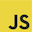So in JavaScript you can make lots of different kinds of objects (even arrays, functions, dates, and regular expressions are objects), but all of these objects have the same type. They have different constructors, but constructors aren’t types.

## Are Types Values?

In JavaScript, the value 100 has the type “number”, but this “number type” itself is not a JavaScript value. Types in JavaScript are extra-lingual. You can ask for an expression’s type, but you’ll just get back a string:

typeof 3 === 'number'
typeof 'hello' === 'string'
typeof { x: 1, y: 2 } === 'object'
typeof typeof 3 === 'string'Ditto for Lua:

type(3) == 'number'
type('hello') == 'string'
type({ x=1, y=2 }) == 'table'
type(type(3)) == 'string'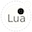In Erlang, you can’t even ask for the type, but there are built-in functions to tell you whether or not an expression has a type:
is_atom(ten).
is_integer($a). is_float(-3.55e-8). is_function(fun (X) -> X*X end). is_reference(make_ref()). is_tuple({dog, "Nika", 5, 'G-SHEP'}). is_list("a string").This is probably a good thing, because as we’ll see, values can have multiple types at the same time. So asking for the type of an expression might be an indication of a type system that is less sophisticated than it could be. In C, you can't even ask or check for a type, but you can see them! The types exist in the code, but they are not values that can be assigned to variables or passed as arguments or returned from functions. Exercise: Try writing C code that uses int as a value. What problems do you run into? In Python, on the other hand, the value 100 has the type int, and int itself is a value in Python. In fact it has the type type. And, you guessed it, type is a value whose type is... yes, type! type(3) == int type('hello') == str type({ 'x':1, 'y':2 }) == dict type(int) == type type(type) == type type(type(type(type(3)))) == type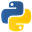Same with Ruby, though in Ruby you tend to see classes more than types: 3.class == Integer 'hello'.class == String {x: 1, y: 2}.class == Hash Integer.class == Class Class.class == ClassJava, too, has objects representing classes: System.out.println(new Integer(3).getClass()); // class java.lang.Integer System.out.println(int.class); // int System.out.println("hello".getClass()); // class java.lang.String System.out.println(new int[]{1, 2, 3}.getClass()); // class [I System.out.println(String.class); // class java.lang.String System.out.println("hi".getClass().getClass()); // class java.lang.Class  Augh, wait, WTF...we have types and now classes? ## Types vs. Classes So what is this thing we call a class? A little hard to define, maybe.... Some languages conflate the notion of type and class, but most people agree there is a difference. TYPECLASS Is all about behaviorIs about both structure and behavior An object can have many typesAn object belongs to exactly one class Is about interfacing and usageIs about construction, and things like fields/properties and methods Example: A string object in Java has multiple types, including String, Comparable, and Object. But only one class: String. String s = "hello"; // "hello" ∈ type String Comparable c = s; // "hello" ∈ type Comparable Object o = s; // "hello" ∈ type Object System.out.println(o.getClass().getName()); // THE class of "hello" is StringIn most languages, declaring a class gives rise to a type. The exceptions to this rule are JavaScript and CoffeeScript, where the word class does not make a type: instead it is a funny way of declaring a function and a prototype. Other languages are similar to Java in having a class construct that makes a type, but they also have ways to make types that “mixin” behaviors, for example Ruby has modules and Swift has protocols. Go has structs for the class-concept and interfaces for the pure behavior-only types. Oversimplying just a bit... A class is an object factory; a type is a behavioral specification. ## Type Expressions In many languages, you get a set of basic types and mechanisms for making new types. Some examples: Language Basic Types Type formers C char, signed char, unsigned char, short, unsigned short, int, unsigned, long, unsigned long, long long, unsigned long long, float, double, long double, _Bool, float _Complex, double _Complex, long double _Complex, float _Imaginary, double _Imaginary, long double _Imaginary enum, *, [], (), union, struct Java boolean, byte, char, short, int, long, float, double interface, class, record, enum, [] Standard ML unit, bool, int, word, real, char, string ->, *, list, option, exn, ref, frag, datatype, {} Python NoneType, NotImplementedType, ellipsis, int, bool, float, complex, str, bytes, tuple, list, bytearray, set, frozenset, dict, function, generator, method, classmethod, staticmethod, module, slice, range, type class Go bool, int8 (byte), int16, int32 (rune), int64, int, uint8, uint16, uint32, uint64, uintptr, float32, float64, complex64, complex128, string, error [], map, *, func, struct, interface, chan Rust bool, i8, i16, i32, i64, i128, isize, u8, u16, u32, u64, u128, usize, f32, f64, char, str, ! (T1, T2), [T ; N], [T], struct, enum, union, ->, &, &mut, *const, *mut, trait, impl Swift Bool, Int8, Int16, Int32, Int64, Int, UInt8, UInt16, UInt32 UInt64, UInt, Float, Double, Character, String, (T1, T2), T?, [T], Set<T>, [K:V], T->U, struct, class Haskell Bool, Int, Integer, Float, Double, Char [a], (a,b), a->b, data So it looks like one of the most common ways to introduce a new type is to use that class notion we saw above. As in Python, for example: >>> class C: pass ... >>> class D: pass ... >>> c, d = C(), D() >>> type(c), type(d), type(c) == type(d) (<class '__main__.C'>, <class '__main__.D'>, False)But something cooler and more computer sciencey are the type operators of the ML-family of languages, like list (for lists), * (for tuples), and -> (for functions): 7 (* int *) (4, "dog") (* int * string *) [5, 3, 2, 7] (* int list *) [ [], [3,3,3], [],  ] (* int list list *) [(3, 4.0),(1, 5.5)] (* (int * real) list *) (5, [2.2, 1.0E~4]) (* int * real list *) fn x => x + 8 (* int -> int *) fn x => fn y => x ^ Int.toString(y) (* string -> int -> string *)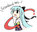Mmmm, so here’s something interesting. SML has type called int list. Does Python? No, in Python, the type is just called list. So [1, "hello"] is legal in Python but illegal in SML. SML has parameterized types. ## Parameterized Types In Standard ML, the types int list and string list and ((int * string) -> bool) list are all different, but they are all instances of the parameterized type 'a list. Lots of languages are like this. Here are some simple examples: LanguageExample Parameterized TypeExample Instantiated TypeNotes Standard ML'a liststring listType variables are 'a, 'b, 'c and so on. If the instantiating type must admit equality, then we write ''a, ''b, ''c, and so on. 'a * 'bstring * bool 'a -> 'b -> 'aint->(bool*int)->int Haskell[a] a->b List Int Int->String Types begin with a capital letter and type variables with a lowercase letter. JavaList<T>List<String> SwiftSet<T>Set<String> By the way, type parameters do not have to be just a simple type variable. ## Type Equivalence When are two types the same? typedef struct { int a; int b; } Point; typedef struct { int a; int b; } Pair; Point x; Pair y;Do x and y have the same type? Should we say “yes” (because they have the same structure), or “no” (because their types have different names, and furthermore appear in different declarations)? These two approaches to determining whether types are the same are structural and named equivalence: Structural Equivalence Name Equivalence Check equivalence by expanding structures all the way down to basic types Strict: Every type declaration defines a new type Loose: Factor out aliases Here is an example from Michael L. Scott: type alink = pointer to cell; subtype blink = alink; p, q : pointer to cell; r : alink; s : blink; t : pointer to cell; u : alink; -- If structural: [p q r s t u] -- If strict name: [p q] [r u] [s] [t] -- If loose name: [p q] [r s u] [t]  Loose name equivalence is pretty common: we like to distinguish things like Point and Pair above but want the flexibility of aliasing. In Ada we can do both explicitly: type Height is new Integer; type Weight is new Integer; -- Can’t add heights and weights subtype Height is Integer; subtype Weight is Integer; -- Now you can freely mix them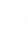ML seems to use structural equivalence: type pair = int * int; type point = int * int; val x: point = (1, 4); val y: pair = x; type person = {name: string, age: int}; type school = {name: string, age: int}; val p: person = {name="Alice", age=22}; val s: school = p;But that’s because type does not define a new type! Only a datatype or abstype declaration creates a new type. abstype person = P of string * int with fun new_person (s, i) = P(s, i) fun name (P(s, i)) = s fun age (P(s, i)) = i end;Exercise: Read this excellent little article that explains name and structural equivalence, with some intersting facts about type equivalence in C. ## Type Compatibility When can a value of type$S$be used in a context that expects type$T$? Possible answers: • When$S$and$T$are equivalent (the same type) • When$S$is a subtype of$T$(where$S$extends or implements$T$) • When$T$is a sum type and$S$is one of the addends • When$S$is an intersection type and$T$is one of the types being intersected • When$S$is$S_1\rightarrow S_2$and$T$is$T_1\rightarrow T_2$and a$T_1$can be used in a$S_1$context and an$S_2$can be used in a$T_2$context • When an$S$can be coerced to a$T$If an$S$can be used where a$T$is expected, we say$S$is compatible with$T. By the way, what is coercion? In: int a; float b; float c; c = a + b;  We may ask whether this is: • An error, fixable by writing c = int_to_float(a) + b? • Allowable? A language that allows this is said to coerce ints to floats, and we say "int is compatible with float". • Ada, Modula, ML: no coercion • Fortran: lots of coercion • C: lots of coercion in numeric types • C++: user can define coercions, even accidentally! Important definitions: Type Conversion Explicit operation that takes in an object of one type and returns an object of a different type that has the "same value" (not necessarily the same bit pattern). Type Coercion Implicit Non-converting Type Cast "Reinterpret" an object as an object of another type by preserving its bit pattern, regardless of value. Activity. Let’s think about the rules. Suppose we are given (I’m using TypeScript notation here):  class Animal { ... } class Dog extends Animal { ... } class Canine = Dog | Wolf class Famous = Notable & Popular  Then we should be able to assign a: • Dog to an Animal • Dog to a Canine • Notable to a Famous • (Dog)=>Dog to a (Dog)=>Animal • (Animal)=>Dog to a (Dog)=>Dog • (Animal)=>Animal to a (Dog)=>Animal • (Dog)=>Animal to a (Dog)=>Animal only but none of these work the other way around. Exercise: Work out in detail why those rules for function compatibility make sense! They are probably not intuitive at first glance. (The rules for function types might look tricky at first. But notice that when passing a function f to a function g, f’s argument type can be a supertype of g’s argument type, while f’s return type can be a subtype of g’s return type. Think about why this must be.) ## Type Inference How do we determine the type of an expression? ML seems to do it the most general way possible. It looks at all the types of the primitive expressions, then at the types of arguments that the subroutines and operators expect, and work your way out to the whole expression. 1 fun fib (n) = 2 let fun fib_helper (f1, f2, i) = 3 if i = n then f2 4 else fib_helper (f2, f1+f2, i+1) 5 in 6 fib_helper (0, 1, 0) 7 end;  • i is int, because it is added to 1 at line 4 • n is int, because it is compared to i at line 3 • all three args at line 6 are int consts, and that’s the only use of fib_helper (given scope of let), so f1 and f2 are int • also 3rd arg is consistent with known int type of i (good!) • and the types of the arguments to the recursive call at line 4 are similarly consistent • since fib_helper returns f2 (known to be int) at line 3, the result of the call at line 6 will be int • Since fib immediately returns this result as its own result, the return type of fib is int If there’s not enough information to resolve to a known type, ML’s inferencer will bring in type variables. Note how clever it is: fun I x = x; 'a -> 'a fun p x y = y 'a -> 'b -> 'b fun first (x, y) = x; 'a * 'b -> 'a fun q x = (hd o hd) x; 'a list list -> 'a fun c x y = if x = y then "y" else "n"; ''a -> ''a -> string  In ML, a type variable beginning with two primes can only be instantiated with a type that admits equality. ML’s inferencing is more powerful than that of other languages, Go, Rust, and Scala require that you put types on parameters. scala> var x = 3; x: Int = 3 scala> var y = x + 3; y: Int = 6 scala> def f(x) = x + 3; <console>:1: error: ':' expected but ')' found. def f(x) = x + 3; ^ scala> def f(x:Int) = x + 3; f: (x: Int)Int  Exercise: Two things complicate type inference are overloading and coercion. Give concrete examples of complications that arise for each. ## Type Checking What if you use an expression in a manner inconsistent with its type, like trying to compute the age of a string (instead of a person). Should the evaluation result in an error, or return a "best guess"? ### Strong Typing vs. Weak Typing Strong TypingWeak Typing Type clashes among unrelated types result in errors. they may be compile time, or even run time (e.g. NoSuchMethodError, or ClassCastException) but they are errors. Type clashes don’t really exist... the language implementation will try to cast the argument into some reasonable (!) type and carry out the operation. Alternate definition: you can’t really circumvent the type system. Example: In Java, even if you explicitly add a cast to cast a string to an int, you get an error. Alternate definition: you can easily circumvent the type system. Example: In C++, you can cast a pointer to an int to a void*, then to a string*, and you get away with it. Some people would argue strong vs. weak typing isn’t a terribly useful thing to worry about. the static/dynamic dimension is a bigger deal. ### Static Typing vs. Dynamic Typing Static TypingDynamic Typing Type checking done at compile time Once a variable’s type is known, it can only be assigned expressions of that type (including related types that can be coerced to it, of course). Type checking done at run time Because checking is deferred until run-time a variable can be assigned an expression of one type and then later an expression of another type. var x; x = 2; print x + 5; x = "dog"; print concat(x, "house");  It is common for languages to have some static typing and some dynamic typing. ### Manifest Typing vs. Implicit Typing Manifest TypingImplicit Typing Types of variables must be given in their declarations. void f(int x, list<int> z) { int y = x * x; z.push_back(y); }  the types of variables will be inferred from context fun f x z = let y = x * x in z @ [y] end  x must be an int because of the "*" operator, so y must be an int as well, and then z must be an int list, and finally f must be int -> int list -> int list. ### What about some popular languages? • Common Lisp: strong, dynamic, implicit • Ada: strong, static, manifest • Pascal: strong, almost static, manifest • Java: strong, some static and some dynamic, manifest • ML: strong, static, implicit • JavaScript and Perl: weak, dynamic • Ruby and Python: strong, dynamic Exercise: Classify Classic Lisp, Smalltalk, Go, and Rust ### Declarations and Static Typing In order to do static typing, you must sometimes specify types when declaring variables, functions, parameters, etc. How is this done? Exercise: Rewrite the examples in the C and Go comparision article in Rust. ### Type Checking with Type Variables Suppose f has type 'a * int -> 'b * 'b -> string. then the following expressions are legal  f(4, 5)("sd","de") f(1,1)(2,2) f([],5)([],[4,4])  but these are not  f(3,2)(4,"p") f((3,2),5)([5.5,2.2],[1,6])  ### Further Reading the literature on strong/weak, static/dynamic, and manifest/implicit typing is enormous, as are the debates and flame wars. Some good reading: ## Dependent Types A dependent type is a type whose definition depends on a value. Examples: • Arrays of length n • Integers less than 8 • Pairs of integers that sum to 21 Does C++ have dependent types? I mean, you can do: template <int n> class BoundedList { public: int contents[n]; }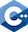but all types that instantiate this template are created at compile time. Languages that fully support dynamic dependent types can eliminate many logic errors at compile time! (The type system essentially lets you formulate compile-time proofs that a program will have a certain run time behavior, in terms of the values it will produce.) Start your study of dependent types at this Stack Overflow question then go to Wikipedia. ## Overview of Common Types ### Numeric Types Lots of variety here: • Integer or Floating point or fixed point or ratios • Integer: Signed or unsigned • Integer: Saturated or unsaturated • Integer: Fixed size (8, 16, 32, 64, 128) or unbounded ("BigInt") • Ratios are also called Rationals • Some languages have a particular kind of ratio type called Decimal • Floats: by size (32, 64, 80, 128) ### Enumerations In type theory, an enumeration is a type with a fixed number of distinct values, ordered from smallest to largest. Typical examples are: • type TrafficLight is (RED, AMBER, GREEN) • type Direction is (NORTH, EAST, SOUTH, WEST) The Boolean type is an enum of the values False and True. Many languages have a character type which is essentially an enumeration. Each character’s "value" is its code point. ### Ranges Some languages allow you to make types such as: • range(1, 100) • range(3, 900, 5) • 1..100 • 1...100 • 1..<100 ### Sum Types and Product Types Suppose we have two types, Boolean and Byte, where: • Boolean has the values {false, true} • Byte has the values {-128, -127, ... 126, 127} Now we form new types: • Boolean + Byte = {false, true, -128, -127, -126, ..., 126, 127} • Boolean × Byte = {(false, -128), (false, -127), ... (false, 127), (true, -128), (true, -127), ..., true(true, 127} Note Boolean has 2 values, Byte has 256 values. Boolean + Byte has 2+256 = 258 values, and Boolean × Byte has 2 * 256 = 512 values. Boolean + Byte is a sum type and Boolean × Byte is a product type. Product types are very common; Python calls them tuples. Haskell and ML just let you write the product type name directly, e.g. Int * String. Often sums and products can be tagged. ### Option Types Option types are one solution to the billion dollar mistake. Basically an object of type T? is pretty much the sum type of T and the type containing a single value representing null. ### Records, a.k.a. Structs Tagged product types go by many names: record, struct, object, hash, etc. Values of such types are thought of as key-value pairs. • Named fields, order may or may not matter • Memory layout often contiguous, but may have holes for alignment reasons, with some languages giving you explicit control over this (Ada has pragmas for it) • Compilers may rearrange fields • Usually you can copy but not compare • Copy can be bitwise, shallow, or deep ### Unions • Cheap way to make a type whose instances can have different forms • Also known as variant records • Generally implemented with overlaying (Fortran uses EQUIVALENCE) • Not needed in languages with subclassing (or with a flexible datatype declaration like ML’s) • Language design choice: is the variant part "tagged"? • If not (C is a good example of this), the whole idea of strong typing goes out the window • If it is tagged, several design choices... • the tag could be permanent once set • Changing the tag could invalidate the object • We could prohibit changing just the tag and require a full reassignment (Ada, Algol68)  datatype primary_color = Red | Blue | Green Red primary_color (Red, 4) primary_color * int  ### Arrays Usually by array we mean a collection of elements indexed by integers or some other enumerated type, that has constant time access of any element given its index. Contrast this with a list, which needn’t have constant time access, and with a map (a.k.a dictionary or associative array), which can be indexed by anything, not just integers or related enumerated types. #### Array Bounds • Normally, array "types" are unbounded, but array instances have bounds. • Is accessing an array outside of its bounds an error? Or does the array object expand to accommodate? • C doesn’t really have arrays! C "arrays" are just pointers and don’t know about bounds or not. Use at your own risk, and be careful! • If the bounds are not known at compile time, we generally make use of a dope vector. (Note that with dope vectors, like any other indirect storage mechanism, watch out for shallow vs. deep copy and equality testing.) • If the bounds are known at compile time, compilers can generate code for element access that uses simple algebraic transforms to compute as much up-front (at compile time) as possible #### Lifetime and Shape Static Bounds Bounds Set at Elaboration Bounds Can be Changed (Dynamic Shape) Global Lifetime C globals Local Lifetime C locals Ada locals Arbitrary Lifetime Java Perl, APL • Local lifetime / Bounds set at elaboration category is interesting: one can use the alloca syscall to implement these, giving you automatic dealloaction on block exit. #### Implementation • Column Major • Row Major (allows 2-D arrays to be the same as arrays of arrays) • Row Pointers — necessary for ragged arrays, good on machines with small segments. #### Slices Several languages (Perl, APL, Fortran 90) have nice syntax for slices. Fortran 90 allows (these examples are from Scott): matrix(3:6, 4:7) columns 3-6, rows 4-7 matrix(6:, 5) columns 6-end, row 5 matrix(:4, 2:8:2) columns 1-4, every other row from 2-8 matrix(:, /2, 5, 9/) all columns, rows 2, 5, and 9  Exercise: Give the equivalent expressions in Julia. Exercise: How are these represented in Python’s numpy library? Good to know: Go uses the term “slice“ to mean something else. ### Strings Most (all?) languages have them, but care is needed: • Are often built-in • Usually have easy to use literal forms, with escape characters • May be immutable • May be interchangeable with character arrays • If mutable, are usually resizable (except in old Pascal • May or may not allow newlines or control characters • Interpolation may or may not be supported • Length is ill-defined! (Number of bytes? number of characters? number of code points? number of graphemes? • Indexing may also be difficult ### Sets Mathematically, a set is an unordered collection of unique elements. • Usually aren’t built-in but most libraries have them • May be mutable or immutable (Python has set and frozenset • Implemented with search trees, tries, hashtables or bitsets ### Pointers A pointer is, more or less, an object through which you reference some other object. They appear in system languages and low level languages. int x = 5; int* p = &x; int* q = NULL; int* r = new int; int* s = new int; cout << *p << *r << s; cout << *q; // crash  #### Basics Here are the crucial things to know: • Pointers are used for dynamic, linked data structures. • Pointers are at a higher level of abstraction than addresses since address computation and error checks can be done on the fly, and in some languages you can overload the dereference operators. • In some languages, pointers can refer only to heap-allocated objects. • In many languages (Java, LISP, ML) pointers are implicit. Example from Lisp: (R (X () ()) (Y (Z ()()) (W ()())))  And from ML: datatype tree = empty | node of 'a * 'a tree * 'a tree; val x_zw = node ('R', node ('X', empty, empty), node ('Y', node ('Z', empty, empty), node ('W', empty, empty)));  #### Reference and Dereference Syntax Can be always explicit, always implicit, or sometimes-implicit (like in Ada).If the pointer is called p (orp in Perl), then

Inthe referent is calledand the field is called
C*p(*p).x or p->x
Adap.allp.all.x or p.x
Modula-2p^p^.x
Javapp.x
Perl%$p$$p{x} or$p->{x}

If the referent is called p (or %p in Perl), then

Inthe pointer is calledand the field is called
C&pp.x
Adap'accessp.x
Perl\%p\$p{x}

#### Memory Leaks and Dangling Pointers

In languages like C requiring explicit allocation and explicit deallocation, it is possible to:

• detach references to heap-allocated objects (memory leak)
• deallocate an objected through a shared pointer (dangling pointer)

How can we prevent such things?

• Prohibit explicit deallocation and use a garbage collector (yeah but this is a cop out sometimes)
• Give programmers some nice tools and conventions, like C++ (this does NOT solve the problem)
• Put in run-time checks to ensure pointers cannot outlive referenced objects (expensive?)
• Put in compile-time checks to ensure pointers cannot outlive referenced objects (a la Rust)

#### Garbage Collection

Covered separately. For now, you can read Wikipedia’s article on garbage collection, and an interesting developerworks article on Java performance and garbage collection.

#### Pointers and Arrays in C

Sometimes, these ideas get conflated in C. After all:

e1[e2] is the same as *(e1 + e2)

For definitions these are completely different things:

  int *x;           /* is totally different from: */
int x;

int *a[n];        /* is totally different from: */
int a[n];


But for declarations, at least in parameter declarations, you can blur the distinction:

  void f(int* a) { ... }
void g(int b[]) { ... }


An array of ints, or pointer to an int, can be passed to either.

### Streams

The term stream is a bit overloaded. Abstractly, a stream:

• is an object that can be read from and written to, usually sequentially, but sometimes with random access
• is called, if read-only, called a source; if write-only, a sink
• is an excellent abstraction; in practice streams are attached to memory buffers, network connections, database connections, files, pipes, or a variety of similar things
• can be made by pasting two streams together

Sometimes we use the term stream to refer to files.

In Java, streams are their own thing. They work like the streams described above (they are processed sequentially), but are in a pretty large subsystem of their own.

### Regular Expressions

These will be covered later in separate notes.

### Subroutines

Subroutines are often objects with their own types. We’ll see these later in separate notes on subroutines.

These will be covered later in separate notes on concurrency.

## Orthogonality

If a type system is orthogonal, then no type is more special and capable than others. The questions you want to ask to see whether a system is orthogonal or not are: Can an object of any type:

• be represented as a literal?
• be declared at all?
• have an initializer?
• be assigned to?
• be passed as a parameter?
• be returned from a function?

You might be surprised at how un-orthogonal some language’s type systems are.

A related question is: Are types themselves objects?

• they aren’t in JavaScript, C, C++, Erlang, or Elixir.
• Python has a type called type, so yes there.
• Java, Ruby, and many other languages have a class called Class, so yes there too.

It’s sometimes undesirable, or maybe even impossible, to get true orthogonality, since:

• Expression oriented languages need an empty type and other languages want to ignore them.
• First class subroutines might be difficult to implement or too slow in languages that claim to be fast.
• Some languages allow arrays of anything; others can store only scalars?
• Array indexing really can only be done by discrete types.
• Member, Local, and Anonymous classes in Java don’t act like top-level ones.
• Should blocks of code be values?
• Simple languages just take short cuts: did you know, in Pascal, you can’t return complex objects from functions?

## Type Theory

There is a great deal of theory surrounding our notion of types. Entire books have been written about this theory.

A good place to start on Type Theory is at Wikipedia. The notes you are reading now have focused almost entirely on the more pragmatic issues of types.

You can also read the Type Theory article in the Stanford Encyclopedia of Philosophy.

More on Mathematics and Philosophy

Many philosophers and mathematicians are interested in a field known as the Foundations of Mathematics. There are three theories that try to be THE foundation: Set Theory (generally the ZFC flavor), Type Theory, and Category Theory. They all work okay and are in some sense equally powerful. See this discussion on Philosophy Stack Exchange and the Wikipedia articles on Intuitionistic Type Theory, Homotopy Type Theory, Curry-Howard Isomorphism, and Foundations of Mathematics.

## Summary

We’ve covered:

• Kinds of types
• A Type Algebra
• Type Systems
• Types vs. Classes
• Type Expressions
• Parameterized Types
• Type Equivalence, Compatibility, Inference and Checking
• Dependent Types
• An overview of Common Types
• Type Theory